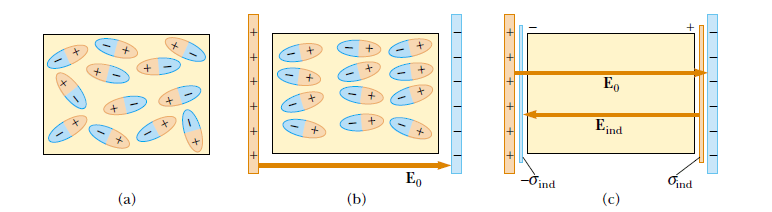# Polarization of a Dielectric between parallel plates of a capacitor

In this post, we will see how Polarization of a Dielectric between parallel plates of a capacitor happens with the help of an external electric field. The potential difference V0 between the plates of a capacitor is reduced to V0/K when a dielectric is introduced. K is the dielectric constant. The potential difference is reduced because the magnitude of the electric field decreases between the plates. In particular, if E0 is the electric field without the dielectric, the field in the presence of a dielectric is E = E0/K.

## Polarize a dielectric with an external electric field

In the next sections, we will see how to polarize a dielectric with an external field regardless of whether the molecules of the dielectric are polar or nonpolar.

### Polarize a dielectric when Dielectric is made up of polar molecules or dipoles

Let us first consider a dielectric made up of polar molecules placed in the electric field between the plates of a capacitor.

The dipoles (that is, the polar molecules making up the dielectric) are randomly oriented in the absence of an electric field, as shown in Figure 1a.

When an external electric field E0 due to charges on the capacitor plates is applied, a torque is exerted on the dipoles, causing them to partially align with the field, as shown in Figure 1b. We can now describe the dielectric as being polarized.figure 1: (a) In the absence of an external electric field Polar molecules are randomly oriented. (b) When an external electric field is applied, the molecules partiallyalign with the electric field. (c) The charged edges of the dielectric can be shown as an extra pair of parallel plates establishing an electric field Eind in the direction opposite to that of external electric field E0.

The degree of alignment of the molecules with the electric field depends on temperature and on the magnitude of the field. In general, the alignment increases with decreasing temperature and with increasing electric field.

### Polarize a dielectric when Dielectric is made up of nonpolar molecules

If the molecules of the dielectric are nonpolar, then the electric field due to the plates produces some charge separation and an induced dipole moment. These induced dipole moments tend to align with the external field, and the dielectric is polarized.

Thus, we can polarize a dielectric with an external field regardless of whether the molecules are polar or nonpolar.

See also  Electric Field due to a Uniformly Charged Spherical Shell & solid sphere
Scroll to top
error: physicsTeacher.in